# RS Aggarwal Solutions Class 10 Chapter 2 Polynomials Ex 2C

In this chapter, we provide RS Aggarwal Solutions for Class 10 Chapter 2 Polynomials Ex 2C Maths for English medium students, Which will very helpful for every student in their exams. Students can download the latest RS Aggarwal Solutions for Class 10 Chapter 2 Polynomials Ex 2C Maths pdf, free RS Aggarwal Solutions Class 10 Chapter 2 Polynomials Ex 2C Maths book pdf download. Now you will get step by step solution to each question.

#### RS Aggarwal Class 10 Solutions Chapter 2 Polynomials Ex 2C

Question 1.
Solution:
Let other zero of x2 – 4x + 1 be a, then
Sum of zeros = −ba = −(−4)1 = 4
But one zero is 2 + √3
Second zero = 4 – (2 + √3) =4 – 2 – √3 = 2 – √3

Question 2.
Solution:
Let f(x) = x2 + x – p(p + 1)
= x2 + (p + 1) x – px – p(p + 1)
= x(x + p + 1) – p(x + p + 1)
= (x + p + 1) (x – p)
Either x + p + 1 = 0, then x = -(p + 1)
or x – p = 0, then x = p
Hence, zeros are p and -(p + 1)

Question 3.
Solution:
p(x) = x2 – 3x – m(m + 3)
= x2 – (m + 3)x + mx – m(m + 3)
= x(x – m – 3) + m(x – m – 3)
= (x – m – 3)(x + m)
Either x – m – 3 = 0, then x = m + 3
or x + m = 0, then x = -m
Zeros are (m + 3), -m

Question 4.
Solution:
a and p are the zeros of a polynomial
and α + β = 6, αβ = 4
Polynomial = x2 – (α + β)x + αβ = x2 – (6)x + 4 = x2 – 6x + 4

Question 5.
Solution:
One zero of kx2 + 3x + k is 2
x = 2 will satisfy it
⇒ k(2)2 + 3 x 2 + k = 0
⇒ 4k + 6 + k= 0
⇒5k + 6 = 0
⇒ 5k = -6
⇒ k = −65
Hence, k = −65

Question 6.
Solution:
3 is a zero of the polynomial 2x2 + x + k
Then 3 will satisfy it
2x2 + x + k = 0
⇒ 2(3)2 + 3 + k = 0
⇒ 18 + 3+ k = 0
⇒ 21 + k = 0
⇒ k = -21
Hence, k = -21

Question 7.
Solution:
-4 is a zero of polynomial x2 – x – (2k + 2)
Then it will satisfy the equation
x2 – x – (2k + 2) = 0
⇒ (-4)2 – (-4) – 2k – 2 = 0
⇒ 16 + 4 – 2k – 2 = 0
⇒ -2k + 18 = 0
⇒ 2k = 18
k = 9

Question 8.
Solution:
1 is a zero of the polynomial ax2 – 3(a – 1)x – 1
Then 1 will satisfy the equation ax2 – 3(a – 1) x – 1 = 0
a(1)2 – 3(a – 1) x 1 – 1 = 0
⇒ a x 1 – 3a + 3 – 1 = 0
⇒ a – 3a + 2 = 0
⇒ -2a + 2 = 0
⇒ 2a = 2
⇒ a = 1

Question 9.
Solution:
-2 is a zero of 3x2 + 4x + 2k
It will satisfy the equation 3x2 + 4x + 2k = 5
3(-2)2 + 4(-2) + 2k = 0
⇒ 3 x 4 + 4(-2) + 2k = 0
⇒ 12 – 8 + 2k = 0
⇒ 4 + 2k=0
⇒ 2k = -4
⇒ k = -2
k = -2

Question 10.
Solution:
Let f(x) = x2 – x – 6
= x2 – 3x + 2x – 6
= x(x – 3) + 2(x – 3)
= (x – 3)(x + 2)
(x – 3)(x + 2) = 0
Either x – 3 = 0, then x = 3
or x + 2 = 0, then x = -2
Zeros are 3, -2

Question 11.
Solution:
Sum of zeros = 1
and polynomial is kx2 – 3x + 5
Sum of zeros = −ba = −(−3)k = 3k
3k = 1
⇒ k = 3
Hence, k = 3

Question 12.
Solution:
Product of zeros of polynomial x2 – 4x + k is 3
Product of zeros = ca
⇒ k1 = 3
⇒ k = 3

Question 13.
Solution:
x + a is a factor of
f(x) = 2x2 + (2a + 5) x + 10
Let x + a = 0, then
Zero of f(x) = -a
Now f(-a) = 2 (-a)2 + (2a + 5)(-a) + 10 = 0
2a2 – 2a2 – 5a + 10 = 0
⇒ 5a = 10
⇒ a = 2

Question 14.
Solution:
(a – b), a, (a + b) are the zeros of 2x3 – 6x2 + 5x – 7
Sum of zeros = −ba
⇒ a – b + a + a + b = −(−6)2
⇒ 3a = 62
⇒ 3a = 3
⇒ a = 1

Question 15.
Solution:
f(x) = x3 + x2 – ax + 6 is divisible by x2 – x
Remainder will be zero
Now dividing f(x) by x2 – x
Remainder = (2 – a) x + b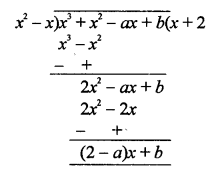(2 – a) x + b = 0
2 – a = 0
⇒ a = 2 and b = 0
Hence, a = 2, b = 0

Question 16.
Solution:
α and β are the zeros of polynomial f(x) = 2x2 + 7x + 5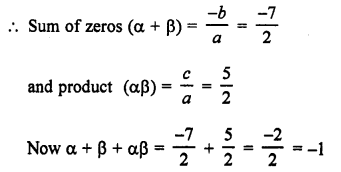Question 17.
Solution:
Division algorithm for polynomials:
If f(x) and g(x) are any two polynomials with g(x) ≠ 0, then we can find polynomial q(x) and r(x).
f(x) = q(x) x g(x) + r(x)
where r (x) = 0
or [degree of r(x) < degree of g(x)]
or Dividend=Quotient x Division + Remainder

Question 18.
Solution:
Sum of zeros = −12
Product of zeros = -3
Polynomial: x2 – (Sum of zeros) x + product of zerosQuestion 19.
Solution: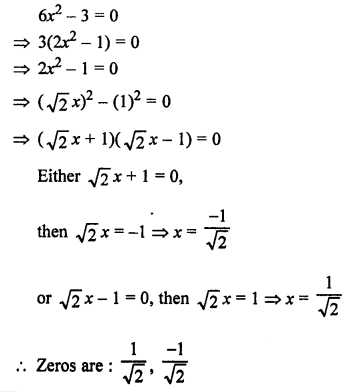Question 20.
Solution:Question 21.
Solution:
α and β are the zeros of polynomial f(x) = x2 – 5x + k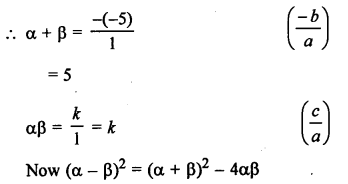(1)2 = (5)2 – 4 k
1 ⇒ 25 – 4k
⇒ 4k = 25 – 1 = 24
Hence, k = 6

Question 22.
Solution: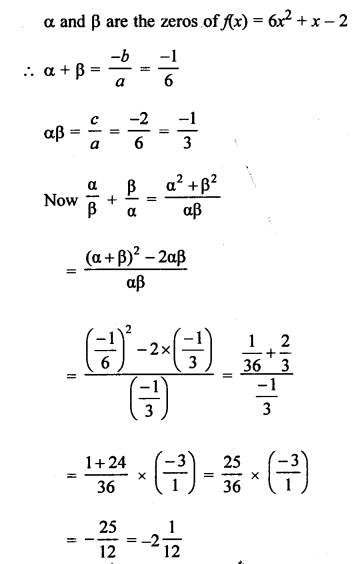Question 23.
Solution:
α and β are the zeros of polynomial
f(x) = 5x2 – 7x + 1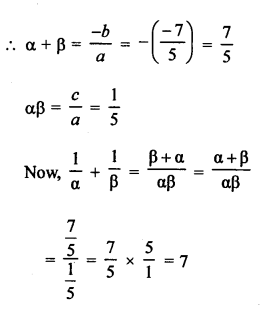Question 24.
Solution:Question 25.
Solution:
(a – b), a and (a + b) are the zeros of the polynomial
f(x) = x3 – 3x2 + x + 1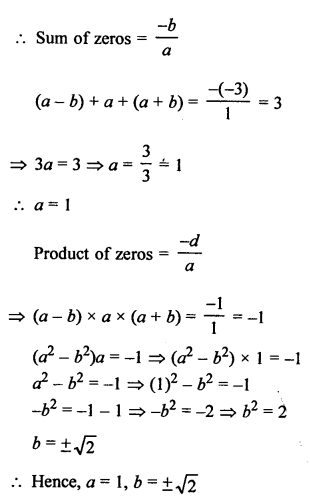All Chapter RS Aggarwal Solutions For Class 10 Maths

—————————————————————————–

All Subject NCERT Exemplar Problems Solutions For Class10

All Subject NCERT Solutions For Class 10

*************************************************

I think you got complete solutions for this chapter. If You have any queries regarding this chapter, please comment on the below section our subject teacher will answer you. We tried our best to give complete solutions so you got good marks in your exam.

If these solutions have helped you, you can also share rsaggarwalsolutions.in to your friends.